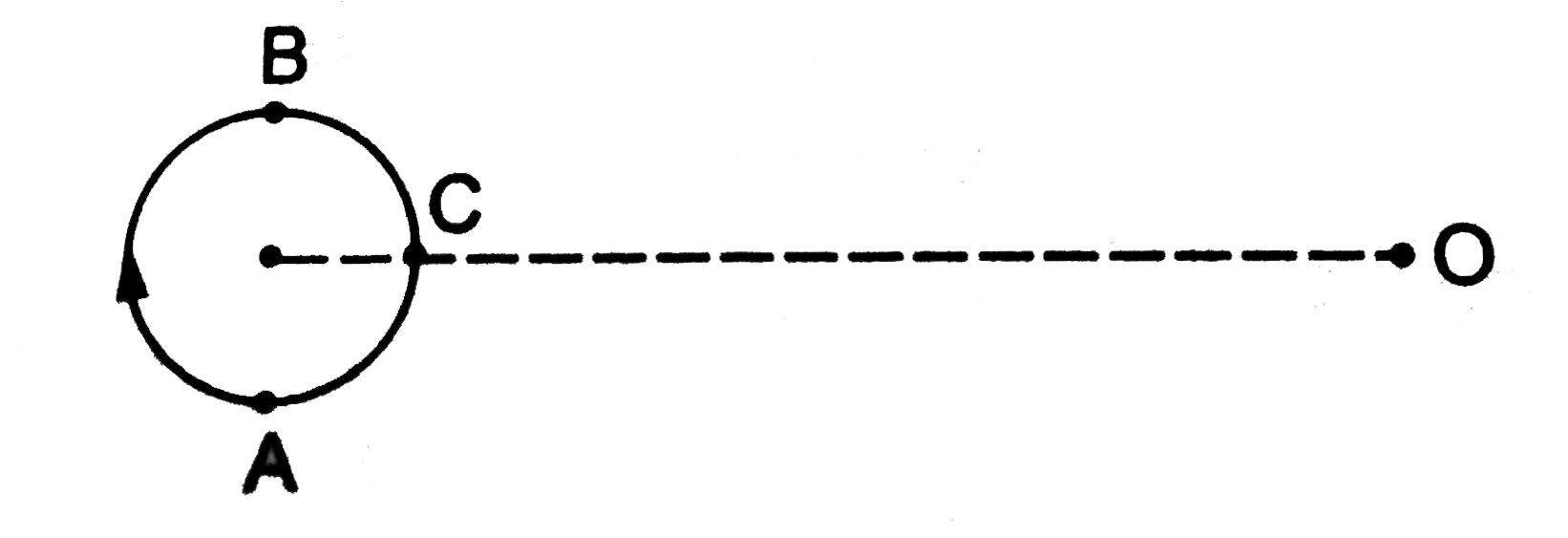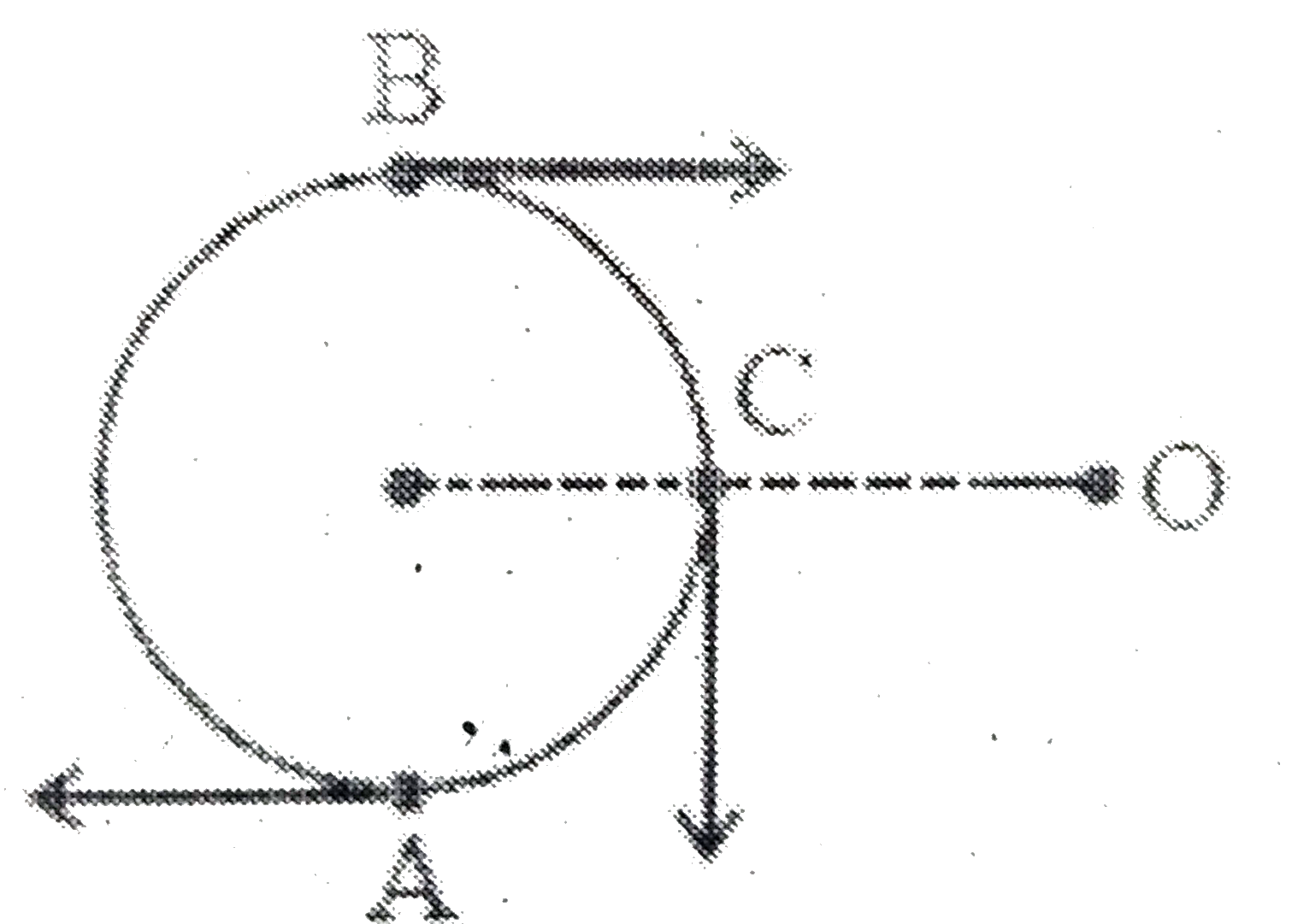# A small source of sound moves on a circle as shown in figure and an observer is sitting at O. Let v_1,v_2,v_3 be the frequencies heard when the sour

24 views
in Physics
closed
A small source of sound moves on a circle as shown in figure and an observer is sitting at O. Let v_1,v_2,v_3 be the frequencies heard when the source is at A,B and C respectively..
A. f _(1) gt f_(2) gt f_(3)
B. f _(1) = f_(2) ft f_(3)
C. f _(2) gt f_(3) fr f_(1)
D.  f_(1) gt f_(3) gt f_(3)

by (93.4k points)
selectedar B : source moving towards observer ltbr at C :S botO
at A : source is moving away from observer